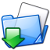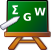# BSE in condensed matter theory

## Error message

Deprecated function: The each() function is deprecated. This message will be suppressed on further calls in node_privacy_byrole_nodeapi_prepare() (line 216 of /var/www/exc/sites/all/modules/node_privacy_byrole/node_privacy_byrole.module).

Many-Body Perturbation Theory1 has been successfully applied in the last three decades, to describe one-particle and two-particle excitations, and still represents a very powerful tool to deal with excitation energies (and today even ground-state total energy) like quasi-particle energies, absorption spectra, collective excitations.

Within many-body perturbation theory one can calculate with a good precision charged excitations (i.e. electron addition and removal energies), using e.g. Hedin's GW approximation2 for the electron self-energy. In the same framework, neutral excitations (e.g. optical and energy-loss spectra) are also well described today through the solution of the Bethe-Salpeter equation (BSE, original article: E.E.Salpeter and H.A.Bethe, Phys. Rev. 84, 1232 (1951)).
An optical absorption experiment creates an interacting electron-hole pair, the exciton. Good agreement between theory and experiment can only be achieved taking into account the electron-hole interaction, especially if the system is a semiconductor or an insulator (small-gap semiconductors and metals, instead, screen this electron-hole interaction, and the resulting contribution can therefore be negligible).
The Bethe-Salpeter couples indeed the electron and the hole, and it has been very successful for the calculation of absorption spectra of a large variety of systems: insulators, semiconductors, atoms, clusters, surfaces or polymers.

The first absorption spectrum within BSE has been obtained by Hanke and Sham3 in 1979. However the first applications of modern ab initio BSE approach date from the 90s4.

The BSE is the third (and last) step of a three-stage rocket, DFT/GW/BSE, whose target is to determine the optical properties of material systems. The first stage, the DFT level, permits one to obtain a ground state structure of the system, by solving the Kohn-Sham (KS) equations, often in Local Density Approximation (LDA). In the second stage, the Quasi-Particle (QP) band structure energies are obtained from the solution of an equation similar to the KS one, but with the KS exchange-correlation potential $V_{xc}$ replaced by the electron self-energy $\Sigma$. The latter is most often evaluated using Hedin's GW approximation. In this approximation $\Sigma$ is the product of the one-particle Green's function G and the screened Coulomb interaction W, calculated in the RPA. The resulting band structures, and in particular the band gap, are generally much closer to the measured ones than the KS results5.

In order to get improved response functions, the electron-hole interaction can then be included by adding the so-called vertex corrections'' beyond the RPA, which is done in practice by solving the four-point Bethe-Salpeter equation (BSE, the third stage) for the polarizability P; this leads in general to excellent absorption and electron energy-loss spectra 6. In particular, one correctly describes the important excitonic effects.

The very ultimate goal of BSE is to find a value for the macroscopic dielectric function $\varepsilon_M (\omega)$, which is directly related with some measurable quantity. The absorption spectrum, e.g., is given by the imaginary part of $\varepsilon_M (\omega)$. If we only consider the resonant part of the excitonic Hamiltonian, i.e we permit only positive frequency transitions to mix, the macroscopic dielectric function is given by
$$\varepsilon_M(\omega)=1-\lim_{{\bf q} \to 0} v_0({\bf q}) \sum_{\lambda} {{\left| \sum_{(n_1n_2)} \left\langle n_1 |e^{-i{\bf q}\cdot{\bf r}}|n_2\right\rangle A_{\lambda}^{(n_1n_2)}\right|^2} \over {E_{\lambda}^{exc}-\omega -i\eta}},$$
where $A_{\lambda}^{(n_1n_2)},E_{\lambda}^{exc}$ are the eigenvectors and eigenvalues of the (resonant) two-particle Hamiltonian:
$$H^{2p,reso}_{(n_{1}n_{2})(n_{3}n_{4})} A_{\lambda}^{(n_3n_4)}=E_{\lambda}^{exc} A_{\lambda}^{(n_1n_2)}.$$
We want now to specify all the ingredients and the approximations occurring in the method. In practice we evaluate everything in frequency domain, reciprocal space and transitions (or orbital) basis. The excitonic Hamiltonian reads $$H_{(vc{\bf k})(v'c'{\bf k}')}^{reso} = \left( E_{c{\bf k}}-E_{v{\bf k}} \right)\delta_{vv'} \delta_{cc'} \delta_{{\bf k}{\bf k}'} + 2v_{vc{\bf k}}^{v'c'{\bf k}'} - W_{vc{\bf k}}^{v'c'{\bf k}'}$$
with (vck) indexes of valence band, conduction band and k-vector, respectively. The spin has been summed up. This Hamiltonian is composed by three parts: a diagonal part that contains the
quasi-particle energies (calculated in the GW approximation) $$H_{(vc{\bf k})(vc{\bf k})}^{diag}= \left( E_{c{\bf k}}-E_{v{\bf k}} \right)\delta_{vv'} \delta_{cc'} \delta_{{\bf k}{\bf k}'},$$
the electron-hole exchange $$H_{(vc{\bf k})(v'c'{\bf k}')}^{exch} = 2{4\pi \over \Omega}\sum_{\bf G\ne 0} {1 \over {|\bf G|^2}} \left\langle c{\bf k} \left|e^{i{\bf G}\cdot {\bf r}}\right| v{\bf k} \right\rangle \left\langle v'{\bf k}' \left|e^{-i{\bf G}\cdot{\bf r}}\right| c'{\bf k}' \right\rangle,$$
and the screened electron-hole interaction part $$H_{(vc{\bf k})(v'c'{\bf k}')}^{scr} = -{4\pi \over \Omega}\sum_{\bf GG'} {\varepsilon^{-1}_{\bf GG'}({\bf q}) \over {|{\bf q} +\bf G|^2}} \left\langle c{\bf k} \left|e^{i({\bf q}+{\bf G})\cdot {\bf r}}\right| c'{\bf k}' \right\rangle \left\langle v'{\bf k}' \left|e^{-i({\bf q} +{\bf G})\cdot{\bf r}}\right| v{\bf k} \right\rangle \delta_{{\bf q},{\bf k}-{\bf k}'}.$$

To summarize, the ingredients for a BSE calculations are:

• ground state structure, in order to have the lda wavefunctions $\left|n{\bf k} \right\rangle$ and eigenvalues (these quantities are contained in the kss file, an output file of the Abinit program)
• GW corrected energies (contained in the gw file, which can be obtained with Abinit as well, or with LSI-GW code)
• the screening matrix $\varepsilon^{-1}_{\bf GG'}({\bf q})$ (contained in the em1 file, still an output file of Abinit)

##### Footnotes

 A.A.Abrikosov, L.P.Gorkov, I.E.Dzyaloshinski, Methods of Quantum Field Theory in Statistical Physics, Prentice-Hall, Englewood Cliffs, NJ (1963)

 L.Hedin Phys. Rev. 139, A796 (1965)

 W.Hanke and L.J.Sham, Phys. Rev. B 21, 4656 (1980).

 G.Onida, L.Reining, .W.Godby, R.Del Sole and W.Andreoni, Phys. Rev. Lett. 75, 818 (1995).
S. Albrecht, G. Onida, and L. Reining, Phys. Rev. B 55, 10278 (1997).
S. Albrecht, L. Reining, R. D. Sole, and G. Onida, Phys. Rev. Lett. 80, 4510 (1998).
L. X. Benedict, E. L. Shirley, and R. B. Bohn, Phys. Rev. Lett. 80, 4514 (1998).
M. Rohlfing and S. G. Louie, Phys. Rev. Lett. 81, 2312 (1998).

 W.J.Aulbur, L.Jonsson and J.W.Wilkins, Solid State Physics 54, 1 (1999)

 G.Onida, L.Reining and A.Rubio, Rev. Mod. Phys. 74, 601 (2002)Body: The new version of EXC is available. In particular the finite momentum transfer has been implemented.Body: A new version of the code has been released: v3.1. Few changes and some bug corrections for this intermediate release.Body: A new version of the code has been released: v3.0.Body: Electronic excitations are probed by experimental techniques such as optical absorption, EELS and photo-emission (direct or inverse).Body: Theoretical Spectroscopy Lectures: Theory and Codes